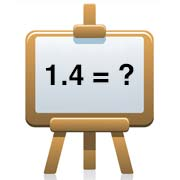Calc

# Decimal to Fraction CalculatorUse this calculator to convert a decimal number to a fraction. To convert a number with repeating decimals, enter the number of trailing decimal places (digits from the end of the number) to repeat. Further down the page, you'll find a guide on how to convert a decimal to a fraction.

## Convert a decimal to a fraction

#### Disclaimer

Whilst every effort has been made in building this decimal to fraction calculator, we are not to be held liable for any special, incidental, indirect or consequential damages or monetary losses of any kind arising out of or in connection with the use of the converter tools and information derived from the web site. This decimal to fraction calculator is here purely as a service to you, please use it at your own risk. Do not use calculations for anything where loss of life, money, property, etc could result from inaccurate conversions.

## How to convert a decimal to a fraction

Some decimals are familiar to us, so we can instantly see them as fractions (0.5 is 1/2, for example). But what of other less obvious decimals - how can you calculate what 0.45, 0.62 or 0.384 is as a fraction, for example? Here's how...

1. Establish whether your decimal is working in tens, hundreds, thousands or more. This will become your multiplier in step 3.
Example: 0.45 is 45 hundredths
2. Write out your decimal as the numerator of a fraction (i.e. above the fraction line). The denominator below the line is always 1, because a decimal is always part of 1.
Example: 0.45/1
3. Multiply your numerator by 10 / 100 / 1000 (your multiplier from step 1), and then do the same for the denominator.
Example: 45/100
4. Simplify your fraction. Find the 'Greatest Common Factor' (the highest number that divides exactly into both the numerator and the denominator).
Example: Both 45 and 100 are multiples of 5, so we can divide both numbers by 5. Result: 9/20.

Should you have a whole number at the beginning of your decimal (6.45), you can simply remove it to work out your decimal, then include it again at the end (Example: 6 and 9/20).

You can go through this process step-by-step in our article on how to convert a decimal to a fraction.

## Why use this decimal to fraction calculator?

Use this converter to check your calculation answers or to get help with figuring out the methodology behind converting a decimal number to a fraction. As well as providing a result for your calculation, we also show you how the answer was achieved.

### Repeating decimals

This decimal to fraction calculator gives you the opportunity to represent repeating decimals by entering a figure into the 'Number of trailing decimal places to repeat' box. Simply enter the number of digits from the end of the decimal to repeat. For other non-repeating decimals, keep the default setting at 0.

As an example, if you want to convert a repeating decimal such as 1.234... then you should enter 1.234 into the Decimal box and 3 into the Number of trailing decimal places to repeat box (signifying that the last 3 digits of the number should repeat).

## Other fraction calculations

Should you wish to add, subtract, multiply, divide or simplify fractions, please see the fractions calculator.

If you have any problems using my decimal to fraction conversion tool, or any suggestions, please contact me.